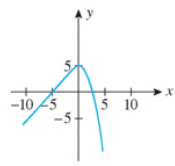Chapter 2.5, Problem 41E

Chapter
Section
Textbook Problem

In Exercises 39-44, determine the values of x, if any, at which each function is discontinuous. At each number where f is discontinuous, state the condition(s) for continuity that are violated.41.f ( x ) = { x + 5 if  x ≤ 0 − x 2 + 5 if  x > 0

To determine
The value of x at which the function is discontinuous.

Explanation

Given information:

The function is,

f(x)={x+5ifx0x2+5ifx>0 (1)

Figure (1)

Definition used:

The function will be continuous when the value of left hand limit and the value of right hand limit is equal to the value of the function at that point and value of that function should satisfy that point.

A function f is continuous at x=a when it follows the following three conditions,

(1) f(a) is defined.

(2) limxaf(x) exists.

(3) limxaf(x)=f(a) .

Calculation:

Substitute 0 for x in equation (1) to find the left hand limit,

limx0f(x)=limx0(x+5)=0+5=5

Substitute 0 for x in equation (1) to find the right hand limit,

limx0+f(x)=lim

Still sussing out bartleby?

Check out a sample textbook solution.

See a sample solution

The Solution to Your Study Problems

Bartleby provides explanations to thousands of textbook problems written by our experts, many with advanced degrees!

Get Started

Simplify the expressions in Exercises 97106. y5/4y3/4

Finite Mathematics and Applied Calculus (MindTap Course List)

Given: NQ bisects MNP,NP=MQ,QP=8,MN=12 Find: NP

Elementary Geometry For College Students, 7e

Solve each equation for if 0360. 2csc=2

Trigonometry (MindTap Course List)

Find the volume of each cylinder:

Elementary Technical Mathematics

Find the matrix A if A=

Finite Mathematics for the Managerial, Life, and Social Sciences